# GMAT Math : Lines

## Example Questions

← Previous 1 3 4 5 6 7 8

### Example Question #1 : Dsq: Calculating The Length Of A Line With Distance Formula

A line segment has an endpoint at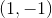; what is its length?

1) Its other endpoint is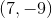2) Its midpoint is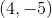BOTH statements TOGETHER are not sufficient to answer the question.
BOTH statements TOGETHER are sufficient to answer the question, but NEITHER statement ALONE is sufficient to answer the question.
Statement 2 ALONE is sufficient to answer the question, but Statement 1 ALONE is not sufficient to answer the question.
EITHER statement ALONE is sufficient to answer the question.
Statement 1 ALONE is sufficient to answer the question, but Statement 2 ALONE is not sufficient to answer the question.
Explanation:

Given the other endpoint, you can use the distance formula to find the length of the segment: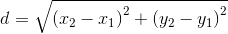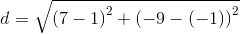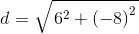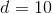Given the midpoint, you can use the distance formula to find the distance from the first endpoint to the midpoint, then double that to get the length of the segment: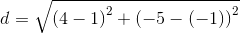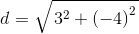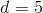The total length is twice that, or 10.

The answer is that either statement alone is sufficient to answer the question.

### Example Question #2 : Dsq: Calculating The Length Of A Line With Distance Formula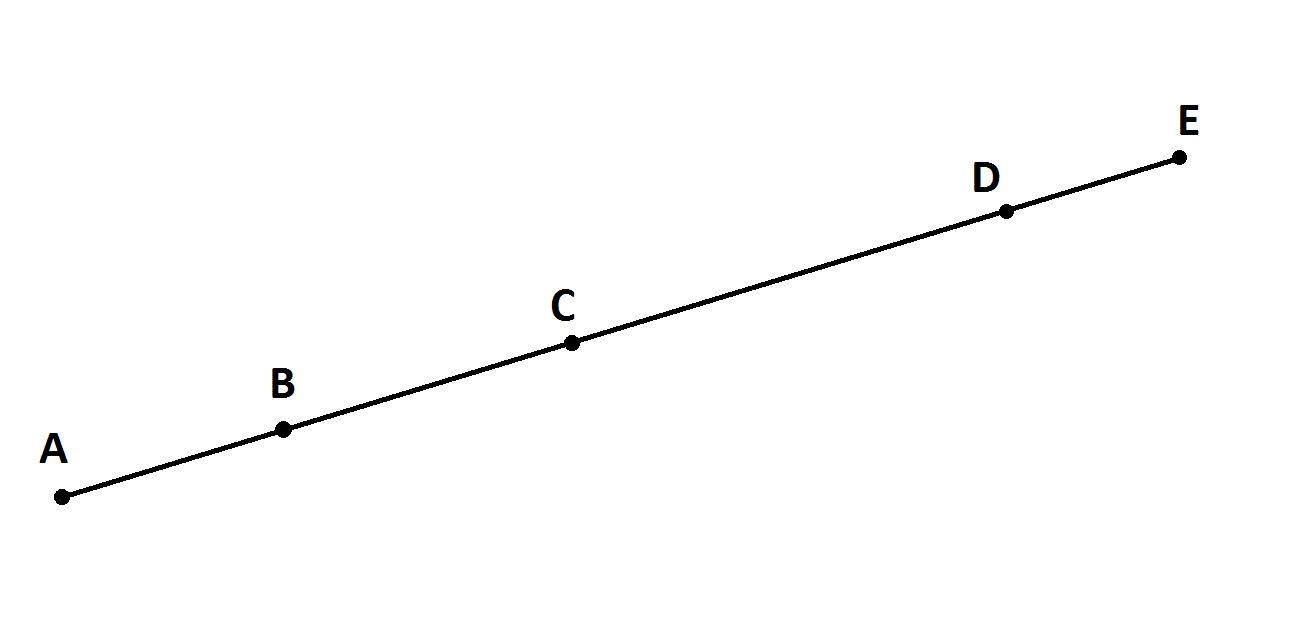Note: Figure NOT drawn to scale.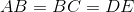Give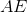.

Statement 1:Statement 2: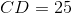Statement 2 ALONE is sufficient to answer the question, but Statement 1 ALONE is NOT sufficient to answer the question.

BOTH statements TOGETHER are sufficient to answer the question, but NEITHER statement ALONE is sufficient to answer the question.

Statement 1 ALONE is sufficient to answer the question, but Statement 2 ALONE is NOT sufficient to answer the question.

BOTH statements TOGETHER are insufficient to answer the question.

EITHER statement ALONE is sufficient to answer the question.

BOTH statements TOGETHER are sufficient to answer the question, but NEITHER statement ALONE is sufficient to answer the question.

Explanation: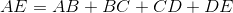If you know only that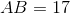, then you know that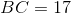and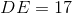, but you still need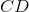, or a way finding it.

If you know only that, you still know only that, but you don't know their actual lengths.

If you know both facts, then you know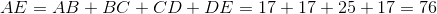### Example Question #3 : Dsq: Calculating The Length Of A Line With Distance Formula

Consider segment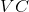.

I) Pointcan be found at the point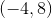.

II) Segmenthad a length ofunits.

Find the coordinates of point.

Either statement alone is sufficient to answer the question.

Statement II is sufficient to answer the question, but statement I is not sufficient to answer the question.

Both statements are necessary to answer the question.

Statement I is sufficient to answer the question, but statement II is not sufficient to answer the question.

Explanation:

Statement I gives us a point.

Statement II gives us the length of the segment.

We are asked to find the coordinates of the other end of the segment. However, we will need more information. Even with all of our information, we have no clue as to the orientation of the line. It could be 14 units straight up and down, it could be a perfectly horizontal line, or something inbetween, thus our answer is:

### Example Question #4 : Dsq: Calculating The Length Of A Line With Distance Formula

Find the length of Segment YZ

I) Point Y is located at the point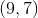.

II) Point Z has a y-coordinate twice that of Point Y and an x-coordinate one-third of Point Y.

Statement I is sufficient to answer the question, but Statement II is not sufficient to answer the question.

Either statement is sufficient to answer the question.

Both statements are needed to answer the question.

Statement II is sufficient to answer the question, but Statement I is not sufficient to answer the question.

Both statements are needed to answer the question.

Explanation:

To find the length of a segment, use the distance formula. The distance formula is given by the following: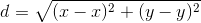Where your's and's correspond to the coordinates of the endpoints.

To find the length of Segment YZ, we need the endpoints.

Statement I gives you Point Y's coordinates.

Statement II relates Point Z's coordinates to Point Y's coordinates. Thus, we can find the point Z using Statement II.

Therefore, we need both.

Recap:

Find the length of Segment YZ

I) Point Y is located at the point.

II) Point Z has a y-coordinate twice that of Point Y, and an x-coordinate one-third of Point Y.

Use Statement II along with Statement I to find the coordinates of Point Z: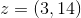Then, use distance formula to find the length of Segment YZ: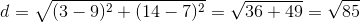### Example Question #5 : Dsq: Calculating The Length Of A Line With Distance Formula

Consider segment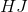I) Endpoint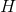is located at the point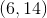.

Ii) Endpoint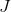has an x-coordinate twice that ofand a y-coordinate 15 times that of H.

What is the length of?

Either statement is sufficient to answer the question.

Statement I is sufficient to answer the question, but statement II is not sufficient to answer the question.

Statement II is sufficient to answer the question, but statement I is not sufficient to answer the question.

Both statements are needed to answer the question.

Both statements are needed to answer the question.

Explanation:

To find the length of a segment, we need both endpoints.

Statement I gives us one endpoint.

Statement II relatesand, allowing us to find the second endpoint.

Thus, we need both. Once we have both endpoints, distance is easily calculated via the distance formula or the Pythagorean theorem.

Using Statement II, we find the second endpoint to be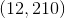. Use the distance formula to find your answer: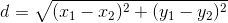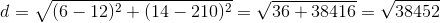### Example Question #1 : Dsq: Calculating The Slope Of A Perpendicular Line

Calculate the slope of a line perpendicular to line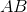.

1. Linepasses through points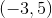and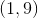.
2. The equation for lineis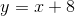.

Both statements taken together are sufficient to answer the question, but neither statement alone is sufficient.

Statements 1 and 2 are not sufficient, and additional data is needed to answer the question.

Statement 1 alone is sufficient, but statement 2 alone is not sufficient to answer the question.

Statement 2 alone is sufficient, but statement 1 alone is not sufficient to answer the question.

Each statement alone is sufficient to answer the question.

Each statement alone is sufficient to answer the question.

Explanation:

Statement 1: We can use the points provided to find the slope of line AB.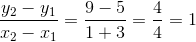Since the slope we're being asked for is of a line perpendicular to line AB, their slopes are inverses of each other.

The slope of our line is then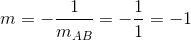Statement 2: Since we're provided with the line's equation, we just need to look for the slope.Whereis the slope andis the y-intercept.

In this case, we haveso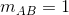. Because our line is perpendicular to line AB, the slope we're looking for is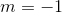### Example Question #2 : Dsq: Calculating The Slope Of A Perpendicular Line

Find the slope of a line perpendicular to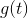.

I)passes through the points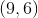and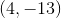.

II)does not pass through the origin.

Both statements are needed to answer the question.

Either statement is sufficient to answer the question.

Statement I is sufficient to answer the question, but statement II is not sufficient to answer the question.

Statement II is sufficient to answer the question, but statement I is not sufficient to answer the question.

Statement I is sufficient to answer the question, but statement II is not sufficient to answer the question.

Explanation:

Find the slope of a line perpendicular to g(t)

I) g(t) passes through the points (9,6) and (4,-13)

II) g(t) does not pass through the origin

Perpendicular lines have opposite reciprocal slopes. For instance: a line with a slope ofwould be perpendicular to a line with slope of.

To find the slope of a line, we just need two points.

I) Gives us two points on g(t). We could find the slope of g(t) and then the slope of any line perpendicular to g(t).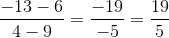So the slope of a line perpendicular to g(t) is equal to: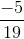II) Is irrelevant or at least not helpful.

### Example Question #3 : Dsq: Calculating The Slope Of A Perpendicular Line

Consider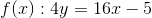and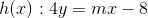.

Find the slope of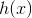.

I)passes through the point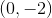.

II)is perpendicular to.

Both statements together are needed to answer the question.

Either statement alone is sufficient to answer the question.

Statement I is sufficient to answer the question, but Statement II is not sufficient to answer the question.

Statement II is sufficient to answer the question, but Statement I is not sufficient to answer the question.

Statement II is sufficient to answer the question, but Statement I is not sufficient to answer the question.

Explanation:

We are given a line, f(x), and asked to find the slope of another line, h(x).

I) Gives a point on h(x). We could plug in the point and solve for our slope. When we do this since x=0 we are unable to find the value for our slope. Therefore, statement I is not sufficient to solve the question.

II) Tells us the two lines are perpendicular. Take the opposite reciprocal of the slope of f(x) to find the slope of h(x).

Therefore,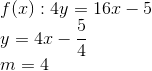and thus the slope of h(x) will be,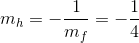.

Statement II is sufficient to answer the question.

### Example Question #1 : Dsq: Calculating The Equation Of A Perpendicular Line

Line AB is perpindicular to Line BC. Find the equation for Line AB.

1. Point B (the intersection of these two lines) is  (2,5).

2. Line BC is parallel to the line y=2x.

Statement 1 alone is sufficient, but statement 2 alone is not sufficient to answer the question.

Both statements taken together are sufficient to answer the question, but neither statement alone is sufficient.

Statements 1 and 2 together are not sufficient.

Statement 2 alone is sufficient, but statement 1 alone is not sufficient to answer the question.

Each statement alone is sufficient.

Both statements taken together are sufficient to answer the question, but neither statement alone is sufficient.

Explanation:

To find the equation of any line, we need 2 pieces of information, the slope of the line and any point on the line. From statement 1, we get a point on Line AB. From statement 2, we get the slope of Line BC. Since we know that AB is perpindicular to BC, we can derive the slope of AB from the slope of BC. Therefore to find the equation of the line, we need the information from both statements.

### Example Question #2 : Dsq: Calculating The Equation Of A Perpendicular Line

Given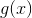, find the equation of, a line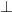to.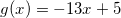I)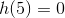.

II) The-intercept ofis at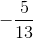.

Statement I is sufficient to answer the question, but statement II is not sufficient to answer the question.

Either statement is sufficient to answer the question.

Statement II is sufficient to answer the question, but statement I is not sufficient to answer the question.

Both statements are needed to answer the question.

Either statement is sufficient to answer the question.

Explanation:

To find the equation of a perpendicular line you need the slope of the line and a point on the line. We can find the slope by knowing g(x).

I) Gives us a point on h(x).

II) Gives us the y-intercept of h(x).

Either of these will be sufficient to find the rest of our equation.

← Previous 1 3 4 5 6 7 8

Tired of practice problems?

Try live online GMAT prep today.